Home MonkeyNotes Printable Notes Digital Library Study Guides Message Boards Study Smart Parents Tips College Planning Test Prep Fun Zone Help / FAQ How to Cite New Title Request

 7.5 Some properties of tangents, secants and chords Theorem: If the tangent to a circle and the radius of the circle intersect they do so at right angles :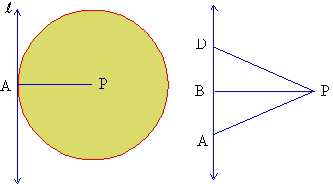Figure 7.9 (a)              Figure 7.9 (b) In figure 7.9 (a) l is a tangent to the circle at A and PA is the radius. To prove that PA is perpendicular to l , assume that it is not. Now, with reference to figure 7.9 (b) drop a perpendicular from P onto l at say B. Let D be a point on l such that B is the midpoint of AD. In figure 7.9 (b) consider D PDB and D PAB seg.BD @ seg.BA ( B is the midpoint of AD) ÐPBD @ ÐPBA ( PB is perpendicular to l ) and seg.PB = seg.PB (same segment) \ D PBD @ D PAB (SAS) \ seg.PD @ seg.PA corresponding sides of congruent triangles are congruent. \ D is definitely a point on the circle because l (seg.PD) = radius. D is also on l which is the tangent. Thus l intersects the circle at two distinct points A and D. This contradicts the definition of a tangent. Hence the assumption that PA is not perpendicular to l is false. Therefore PA is perpendicular to l. Angles formed by intersecting chords, tangent and chord and two secants: If two chords intersect in a circle, the angle they form is half the sum of the intercepted arcs. In the figure 7.10 two chords AB and CD intersect at E to form Ð1 and Ð 2.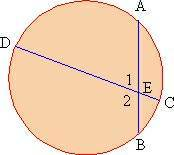Figure 7.10 m Ð1 =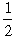(m seg.AD + m seg.BC) and m Ð2 =(m seg.BD + m seg.AC) Tangent Secant Theorem: If a chord intersects the tangent at the point of tangency, the angle it forms is half the measure of the intercepted arc. In the figure 7.11 l is tangent to the circle. Seg.AB which is a chord, intersects it at B which is the point of tangency.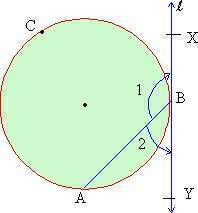Figure 7.11 The angles formed Ð ABX and Ð ABY are half the measures of the arcs they intercept. m Ð 1 =m (arc ACB) m Ð 2 =m (arc AB) This can be proved by considering the three following cases.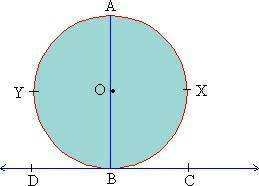Figure 7.12 O is the center of the circle Line DBC is tangent to it at B. BA is the chord in question. X is a point on the circle on the C side of BA and Y is on the D side by BA. Index 7.1 Introduction 7.2 Lines of circle 7.3 Arcs 7.4 Inscribed angels 7.5 Some properties od tangents, secants and chords 7.6 Chords and their arcs 7.7 Segments of chords secants and tangents 7.8 Lengths of arcs and area of sectors Chapter 8
 Search: All Products Books Popular Music Classical Music Video DVD Toys & Games Electronics Software Tools & Hardware Outdoor Living Kitchen & Housewares Camera & Photo Cell Phones Keywords: